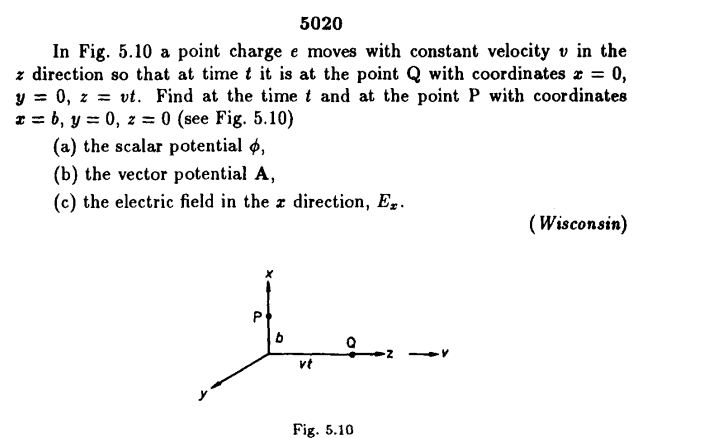# The potential electric and vector potential of a moving charge

• LCSphysicist

#### LCSphysicist

Homework Statement
.
Relevant Equations
.I could try to apply the Liénard-WIechert equations immediatally, but i am not sure if i understand it appropriately, so i tried to find by myself, and would like to know if you agree with me.

When the information arrives in ##P##, the particle will be at ##r##, such that this condition need to be satisfied:
$$\frac{(vt-r)^2}{v^2} = \frac{r^2+b^2}{c^2} (1)$$

So, we have $$\phi = \frac{kq}{s} = \frac{kq}{r}$$
Also, $$\vec A = \frac{\mu}{4\pi} \int \frac{J' dV'}{|r-r'|} = \frac{\mu}{4\pi} \frac{q v}{|r|}$$

such that r satisfies ##(1)##.

Is it right? Do you agree with it? I am asking because i remember to read somewhere that the potential electric not necessarilly need to move at speed of light, but i think this is the case in Lorenz gauge. I haven't assumed any gauge here.

•BvU